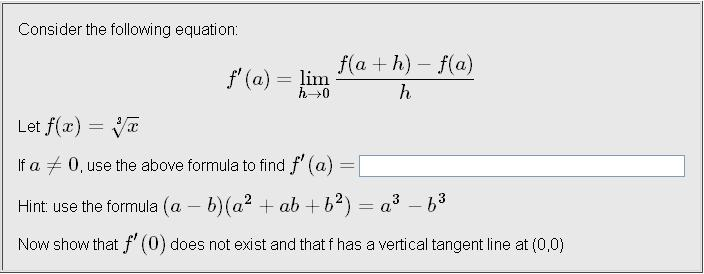# Finding Derivative Using 'Definition of Derivative'

## Homework Statement## Homework Equations

As seen above in question.

## The Attempt at a Solution

Well, I substituted f(x) into the definition of derivative equation and then multiplied the expression by its conjugate, thus getting the expression in the following form:
f'(a) = lim (a + h)2/3 - a2/3 / h [(a + h)1/3 + a1/3]
...h→0

Now, from this point on, it's just algebra and I am having trouble manipulating this expression so that I can divide the "h" from the denominator. I know I am supposed to use the "hint" given in the question but how would I incorporate that into finding the answer?

Thanks.

Staff Emeritus
Homework Helper
Gold Member

## Homework Statement## Homework Equations

As seen above in question.

## The Attempt at a Solution

Well, I substituted f(x) into the definition of derivative equation and then multiplied the expression by its conjugate, thus getting the expression in the following form:
f'(a) = lim (a + h)2/3 - a2/3 / h [(a + h)1/3 + a1/3]
...h→0

Now, from this point on, it's just algebra and I am having trouble manipulating this expression so that I can divide the "h" from the denominator. I know I am supposed to use the "hint" given in the question but how would I incorporate that into finding the answer?

Thanks.
Well, multiplying by the conjugate, doesn't work here because getting a difference of squares isn't helpful when working with the cube root.

Use the hint given.

Let $a=\sqrt{x+h}\,,$ and $b=\sqrt{x}\ .$

I did that but it ended up getting to this expression after some simplifications:
[ (x + h)2/3(x)1/3 - (x + h)2/3(x)1/3 ] / h
as h → 0.

You can already see what the problem is here. The numerator results in 0, which is subsequently divided by h.

Staff Emeritus
You want to multiply $\sqrt{x+h}\,-\,\sqrt{x}$ by something that results in cubing each of those terms. Right?